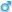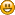###Author Topic: Current and voltage in AC circuits?  (Read 14325 times)

0 Members and 1 Guest are viewing this topic.

####Peter

• New member
•• Posts: 4
• Karma: +0/-0
• Gender:##### Current and voltage in AC circuits?
« on: May 21, 2005, 05:45:20 PM »
I have problems calculating voltage and current in AC circuits.

The circuits: http://img238.echo.cx/img238/5515/ac0sl.gif

Can someone verify and tell me if this is correct? I am pretty shure about circuit 1 and 2, but not the third circuit.

My calculations for circuit:

1a.

f=159Hz
Xc=1/wc=10010ohm

I=sqrt(R^2+Xc^2)  sqrt(10000^2+10010^2)=3,53*10^-4A

UR=I*R  3,53*10^-4*10000=3,53V
UC=I*1/wc  3,53*10^-4*10010=3,53V

U=sqrt(UC^2+UR^2)=5V

2a.

f=159Hz
Xc=10010ohm

I1=U/R  5/10000=5*10^-4A
I2=U/R  5/10010=5*10^-4A

I=I1+I2=10^-3A

3a.

f=159Hz
Xc=10010ohm

Replace the two resistors with: 1/10000+1/10000=1/ans=5000ohm

I=U/sqrt(R^2+(1/wc)^2)  5/sqrt(5000^2+10010^2)=4,47*10^-4A

U=I*R  4,47*10^-4*5000=2,235V

IR1=U/R  2,235/10000= 2,235*10^-4A
IC=U/(1/wc)  2,235/10010=2,23*10^-4A

Thanks in advance!

####peranders

• Administrator
• Reached Nirvana
••• Posts: 1230
• Karma: +6/-0
• Gender:• Forum owner##### Current and voltage in AC circuits?
« Reply #1 on: May 21, 2005, 06:11:02 PM »
The last circuit is 2.5 volts and 5 kohms drivning the cap.
/Per-Anders Sjöström, owner of this forum

Homepage with my DIY hifi stuff

####Peter

• New member
•• Posts: 4
• Karma: +0/-0
• Gender:##### Current and voltage in AC circuits?
« Reply #2 on: May 21, 2005, 07:39:09 PM »
Ok, 2,5V=(5*10k)/(10k+10k)=U0

If the cap gets 2,5V then the resistor R1 also gets 2,5V because they are in parallel, right?

Then,

IR1=U/R  2,5/10000=2,5*10^-4A
IC=U/(1/wC)  2,5/10010=2,498*10^-4A

I=5/sqrt(5000^2+10010^2)=4,47*10^-4A

But I-IR1-IC does not match. What am I doing wrong here?

####peranders

• Administrator
• Reached Nirvana
••• Posts: 1230
• Karma: +6/-0
• Gender:• Forum owner##### Current and voltage in AC circuits?
« Reply #3 on: May 21, 2005, 07:58:38 PM »
Quote from: Peter
What am I doing wrong here?

The voltage over the capacitor is frequency dependent.

The circuit is only a resistor (5 kohms) and a cap.

The capacitor will get 2.5 volts across itself only at DC.

Compare the first circuit in your drawing.
/Per-Anders Sjöström, owner of this forum

Homepage with my DIY hifi stuff

####Peter

• New member
•• Posts: 4
• Karma: +0/-0
• Gender:##### Current and voltage in AC circuits?
« Reply #4 on: May 21, 2005, 09:01:25 PM »
I get,

I=2,5/sqrt(5000^2+10010^2)=2,23*10^-4A

The current will be the same in R and C because they are in serie.

Then,

UR=1,115V and UC=2,23V

Check the voltage sqrt(1,115^2+2,23^2)=2,5V

Seems to be correct. What do you think?

####Peter

• New member
•• Posts: 4
• Karma: +0/-0
• Gender:##### Current and voltage in AC circuits?
« Reply #5 on: May 23, 2005, 06:14:36 PM »
Thank you very much for your help peranders!

####peranders

• Administrator
• Reached Nirvana
••• Posts: 1230
• Karma: +6/-0
• Gender:• Forum owner##### Current and voltage in AC circuits?
« Reply #6 on: May 24, 2005, 08:58:39 AM »
You're welcome.Hint: Norton-Thevenin convertions may be very handy sometimes, also Y-D convertions so I recommend that you study those so you will know about them.
/Per-Anders Sjöström, owner of this forum

Homepage with my DIY hifi stuff